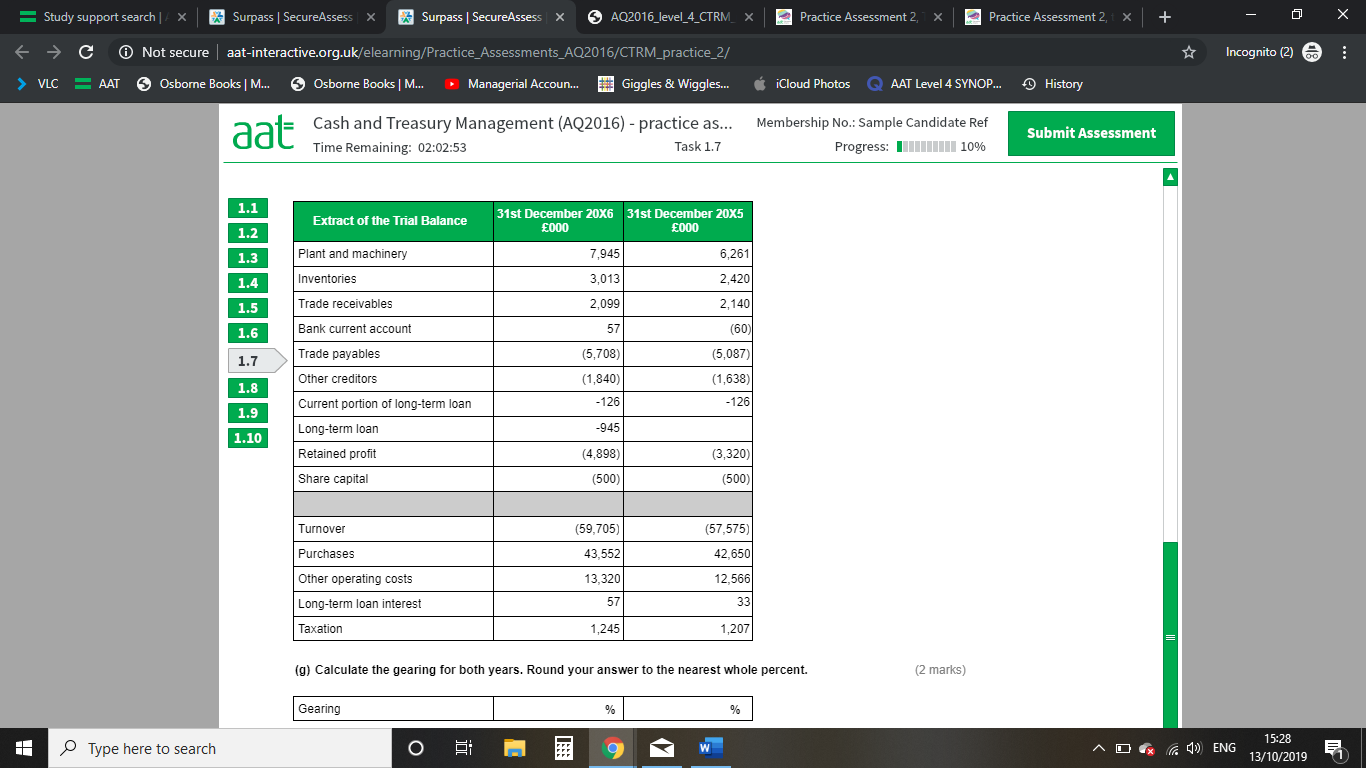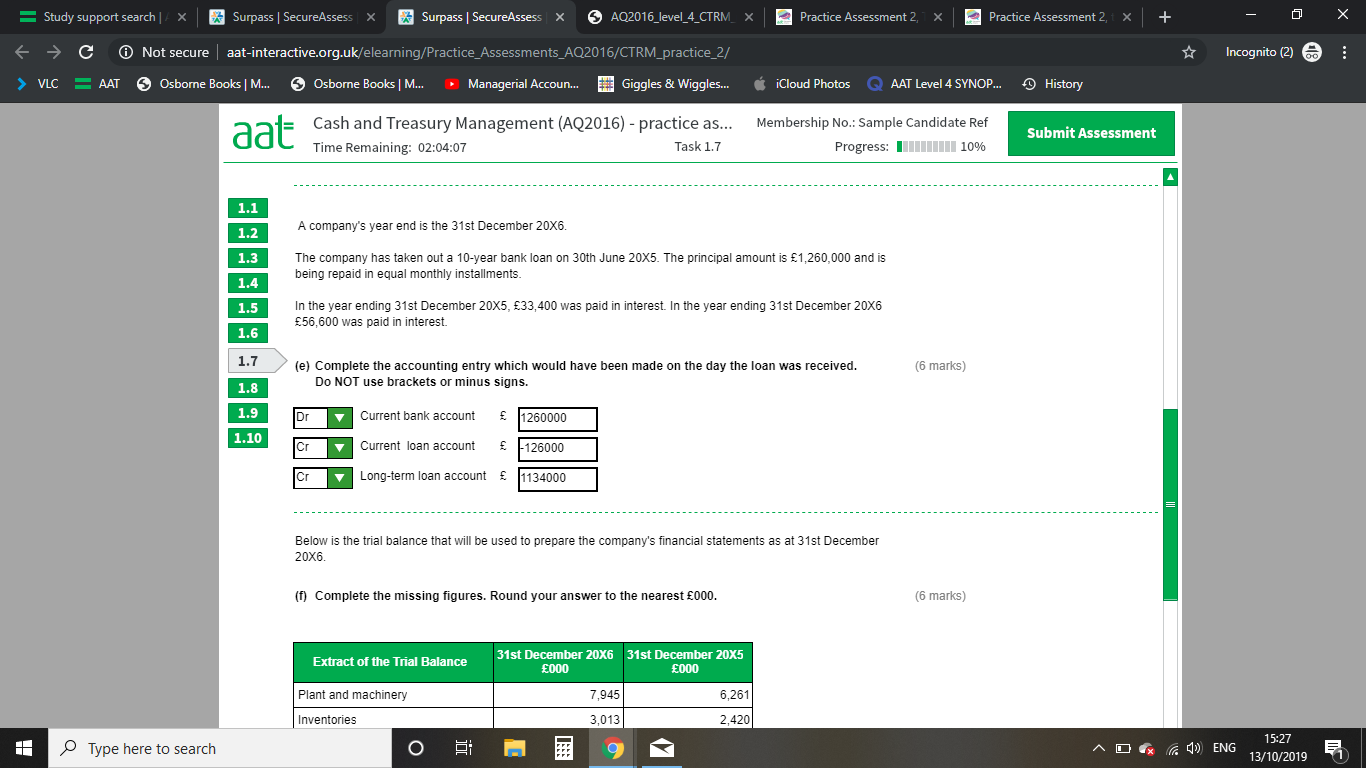# Practice assessment 2, Task 7 F and G, extract of trial balance and Gearing Ratio

I was wondering if someone could help me please, I am really struggling with this one.

So you are given the above trial balance and information. And are asked to fill in 6 blanks.

I understand that the 'Current portion of Long-term Loan' is 10% of 1,260,000 as the loan is over 10 years and is equally portioned

I also understand that 'Long-term Loan' 2016 is £126,000 lower than 2015 figure (as you are paying off £126,000 of the loan per year.
But i cannot for the life of me work out where -£1,071,000 comes from for 2015 'Long-term Loan'

I also understand that 'Long-term loan interest' is 57 and 33 as they are just stated above.

But this 1071 figure i just can't reach. I presume i start off with 1260 as this is the figure of the original loan, but then i cant find the 189k difference. I have tried taking off 126, and then 33 interest (which is what i thought the question was asking) but that would mean i am still 30k out.

Also, question G is work out the gearing ratio for both years, so i understand i would use the formula Total Debt / Total Debt + Equity but i have no idea where i would get these figures from in the above trial balance. The answers are 2016 = 17% and 2015 = 25%

Thank you very much in advance and sorry for rambling on